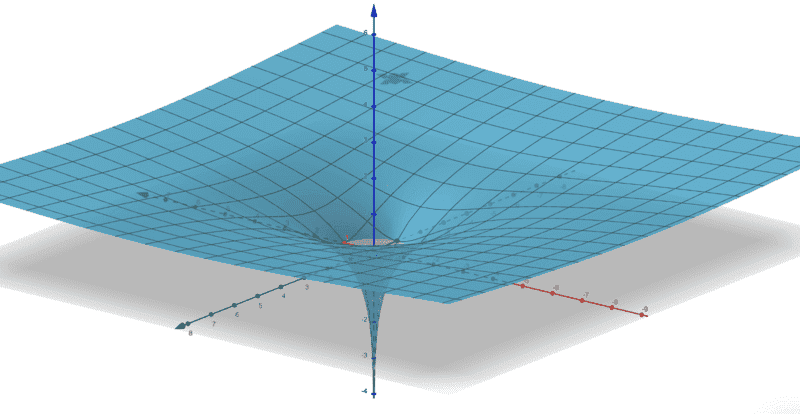# Dimensional confusion with a Lagrangian problem

• I
dyn
Hi
I have been doing a question on Lagrangian mechanics. I have the solution as well but i have a problem with the way the question is asked regarding dimensions.
The 1st part of the question says that a particle of mass m with Cartesian coordinates x , y , z moves under the influence of gravity on the surface

z = (1/2) In(x2+y2)

Now this doesn't seem right because the argument of a log should be dimensionless. Also the log of a number is a dimensionless number so the LHS of the equation has dimensions of length wile the RHS is dimensionless.

Following on from this comes my next confusion ; the Lagrangian which is given in polar coordinates involves a term containing (m/2) multiplied by (r dot )2 multiplied by ( 1 + r-2 ). This is adding 2 terms together with different dimension which is not allowed

Am i right that the question is written incorrectly or have i got something wrong ?

Thanks

•vanhees71

docnet
The 1st part of the question says that a particle of mass m with Cartesian coordinates x , y , z moves under the influence of gravity on the surface

z = (1/2) In(x2+y2)

Now this doesn't seem right because the argument of a log should be dimensionless. Also the log of a number is a dimensionless number so the LHS of the equation has dimensions of length wile the RHS is dimensionless.
I'm not sure if this equation involves any units, as it might just be an equation of a surface. The graph of this equation isI'm guessing that you're expected to use this equation as a constraint somehow

Maybe there is an implicit constant in z, writtens as the log of another constant with dimension length-squared?

E.g. a term like

log(x^2)-log(C)

is dimensionless if [C]=[x^2].

dyn
The equation for z is exactly as given in #1. There are no other constants mentioned

dyn
I'm not sure if this equation involves any units, as it might just be an equation of a surface. The graph of this equation is

View attachment 292140

I'm guessing that you're expected to use this equation as a constraint somehow
There must be units involved because to obtain a Lagrangian all the term involved must have units of energy. The kinetic energy terms involve time derivatives of position coordinates so z must have dimensions of position

Gold Member
2022 Award
You are perfectly right. The equation written is undefined. You cannot have dimensionful quantities as argument of a function, which is not of the form ##f(x)=A x^B##.

•dyn
ergospherical
I don't think this sort of thing (dimensionless co-ordinates) is particularly uncommon, especially in applied maths. You could think of it as ##x = \tilde{x} / u_L##, with ##u_L## a suitable unit of length (e.g. ##1 \mathrm{m}##) and ##\tilde{x}## the usual dimensionful coordinate.

•dyn and docnet
dyn
This example came from a maths exam. Maybe mathematicians aren't as concerned with dimensional analysis as physicists !